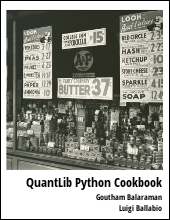Provides an example of valuing bonds with credit spreads using QuantLib Python. This post walks through an example of shifting the yield term structure.

Visit here for other QuantLib Python examples. If you found these posts useful, please take a minute by providing some feedback.

In an earlier example on pricing fixed rate bonds I demonstrated how to construct and value bonds using the given yield curve. In this example, let us take a look at valuing bonds with credit spreads. We will show how to add credit spreads to the give yield curve using different approaches.

As usual, let us start by importing the QuantLib library and pick a valuation date and set the calculation instance evaluation date.

In :
import QuantLib as ql
calc_date = ql.Date(26, 7, 2016)
ql.Settings.instance().evaluationDate = calc_date


For simplicity, let us imagine that the treasury yield curve is flat. This makes it easier to construct the yield curve easily. This also allows us to directly shock the yield curve, and provides a validation for the more general treatment of shocks on yield curve.

In :
flat_rate = ql.SimpleQuote(0.0015)
rate_handle = ql.QuoteHandle(flat_rate)
day_count = ql.Actual360()
calendar = ql.UnitedStates()
ts_yield = ql.FlatForward(calc_date, rate_handle, day_count)
ts_handle = ql.YieldTermStructureHandle(ts_yield)


Now let us construct the bond itself. We do that by first constructing the schedule, and then passing the schedule into the bond.

In :
issue_date = ql.Date(15, 7, 2016)
maturity_date = ql.Date(15, 7, 2021)
tenor = ql.Period(ql.Semiannual)
calendar = ql.UnitedStates()
date_generation = ql.DateGeneration.Backward
month_end = False
schedule = ql.Schedule (issue_date, maturity_date,
tenor, calendar,
bussiness_convention,
bussiness_convention,
date_generation,
month_end)

In :
settlement_days = 2
day_count = ql.Thirty360()
coupon_rate = .03
coupons = [coupon_rate]

# Now lets construct the FixedRateBond
settlement_days = 0
face_value = 100
fixed_rate_bond = ql.FixedRateBond(
settlement_days,
face_value,
schedule,
coupons,
day_count)


Now that we have the fixed_rate_bond object, we can create a DiscountingBondEngine and value the bond.

In :
bond_engine = ql.DiscountingBondEngine(ts_handle)
fixed_rate_bond.setPricingEngine(bond_engine)
fixed_rate_bond.NPV()

Out:
114.18461651948999

So far, we have valued the bond under the treasury yield curve and have not incorporated the credit spreads. Let us assume that the market prices this bond with a 50BP spread on top of the treasury yield curve. Now we can, in this case, directly shock the flat_rate used in the yield term structure. Let us see what the value is:

In :
flat_rate.setValue(0.0065)
fixed_rate_bond.NPV()

Out:
111.5097766266561

Above we shocked the flat_rate and since the yield term structure is an Observer observing the Observable flat_rate, we could just shock the rate, and QuantLib behind the scenes recalculates all the Observers. Though, this approach is not always viable, in cases such as a bootstrapped bond curve. So let us look at two different approaches that can be used. Before we do that, we need to reset the flat_rate back to what it was.

In :
flat_rate.setValue(0.0015)
fixed_rate_bond.NPV()

Out:
114.18461651948999

#### Parallel Shift of the Yield Curve

The whole yield curve can be shifted up and down, and the bond revalued with the help of the ZeroSpreadedTermStructure. The constructor takes the yield curve and the spread as argument.

In :
spread1 = ql.SimpleQuote(0.0050)

fixed_rate_bond.setPricingEngine(bond_engine)

# Finally the price
fixed_rate_bond.NPV()

Out:
111.50977662665609

Once we have constructed the spreaded term structure, it is rather easy to value for other spreads. All we need to do is change the SimpleQuote object spread1 here.

In :
spread1.setValue(0.01)
fixed_rate_bond.NPV()

Out:
108.89999943320038

#### Non-Parallel Shift of the Yield Curve

The above method allows only for parallel shift of the yield curve. The SpreadedLinearZeroInterpolatedTermStructure class allows for non parallel shock. First, let us mimic a parallel shift using this class. For the constructor, we need to pass the yield term structure that we wish to shift, and the a list of spreads and a list of the corresponding dates.

In :
spread21 = ql.SimpleQuote(0.0050)
start_date = calc_date
ts_handle,
[start_date, end_date]
)

fixed_rate_bond.setPricingEngine(bond_engine)

# Finally the price
fixed_rate_bond.NPV()

Out:
111.50977662665609

Here, once again we can change the value of spread2 to value for other shocks.

In :
spread21.setValue(0.01)
fixed_rate_bond.NPV()

Out:
108.89999943320038

We can easily do non-parallel shifts just by shocking one end.

In :
spread21.setValue(0.005)
fixed_rate_bond.NPV()

Out:
111.25358792334083

The SpreadedLinearZeroInterpolatedTermStructure is a very powerful class and can be used to implement key-rate duration calculations.

quantlib   python   finance

Related PostI am Goutham Balaraman, and I explore topics in quantitative finance, programming, and data science. You can follow me @gsbalaraman.

Checkout my bookUpdated posts from this blog and transcripts of Luigi's screencasts on YouTube is compiled into QuantLib Python Cookbook .